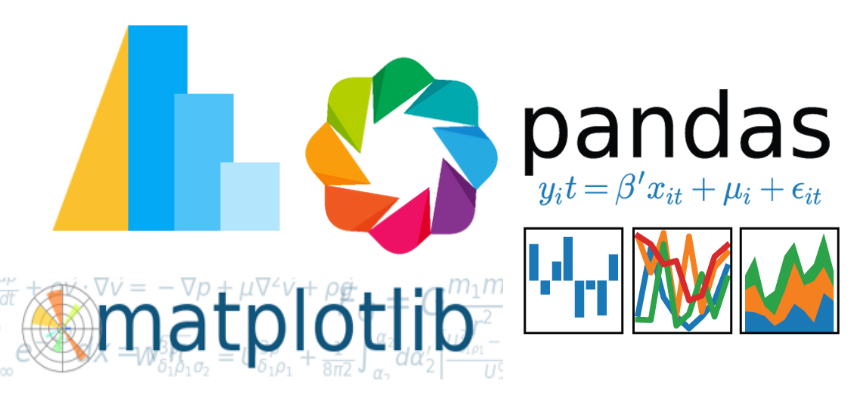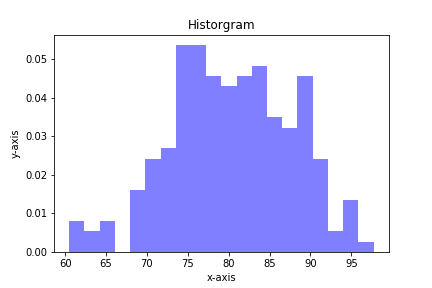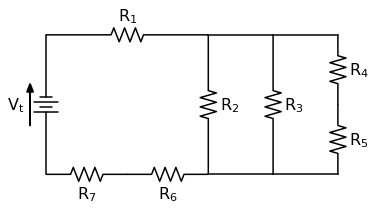Quiver plots using Python, matplotlib and Jupyter notebooksA quiver plot is a type of 2D plot that shows vector lines as arrows. Quiver plots are useful in electrical engineering to visualize electrical potential and valuable in mechanical engineering to show stress gradients. In this post, we will build three quiver plots using Python, matplotlib, numpy, and Jupyter notebooks.

Plotting a stress-strain curve with four libraries: matplotlib, pandas, altair and bokehAfter watching a great webinar about plotting with different python libraries, I wanted to see what it was like to make a stress strain curve using four different modules: pandas, matplotlib, altair and bokeh (with holoviews).

Plotting Histograms with matplotlib and PythonHistograms are a useful type of statistics plot for engineers. A histogram is a type of bar plot that shows the frequency or number of values compared to a set of value ranges. Histogram plots can be created with Python and the plotting package matplotlib. The plt.hist() function creates …

Solving a Circuit Diagram Problem with Python and SchemDraw

In this post we will solve a circuit diagram problem using a Python package called SchemDrawDiffusion Calculation with Python and Pint

I was working on an engineering problem involving diffusion that involved a couple of different units including joules, grams, kilograms, meters, centimeters, moles, megapascals and weight percent. To make sure that I kept track of all the units and unit conversions throughout the problem, I thought I'd try using pint, a Python package for unit conversions.